# Ring torus

(Redirected from Torus in 3-space)

## Definition

The ring torus is a form of embedding of the torus in three-dimensional Euclidean space. This surface type is not unique up to isometry or even up to similarity transformations, but rather, depends on two parameters for a description up to isometry and on one parameter for a description up to similarity transformations.

A ring torus can be defined as the surface obtained by revolving a circle about a line in its plane that does not intersect it.

To describe a ring torus up to isometry, we need two parameters:

• The radius of the circle being revolved, which we call the tube radius and denote by$a$.
• The perpendicular distance from the center of the circle being revolved to the axis of revolution, which we denote by$c$.

The condition that the axis of revolution does not intersect the circle being revolved is equivalent to the condition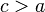$c > a$.

### Implicit and parametric descriptions

Degree of generality Implicit description What the parameters mean Parametric description What the additional parameters mean Comment
Arbitrary Fill this in later
Up to rigid motions (rotations, translations, reflections)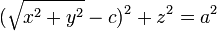$(\sqrt{x^2 + y^2} - c)^2 + z^2 = a^2$, where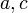$a,c$ are positive and$c > a$.$c$ is the radius of the central circle (spine) of the ring torus, and$a$ is the tube radius of the ring torus. This describes the ring torus where the axis of revolution is the$z$-axis.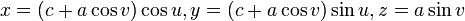$x = (c + a \cos v)\cos u, y = (c + a \cos v)\sin u, z = a \sin v$$u$ is an angle giving local polar coordinates for the point any fixed location of the circle being rotated.$v$ is the angle giving polar coordinates for the center of the circle, on the spine circle.
Up to similarity transformations We could rescale the above to normalize either one of$c$ and$a$ to 1, but we cannot normalize both simultaneously.

## Related surfaces

Note that none of the surfaces below is topologically a torus.

• Horn torus is a related construct where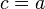$c = a$, i.e., the case of a circle being revolved about its tangent line. This is topologically not even a manifold.
• Spindle torus is a related construct where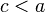$c < a$, i.e., the case of a circle being revolved about a line intersecting it. A spindle torus has an inner and outer surface respectively called a lemon (the surface of revolution of a circular lens) and an apple. The spindle torus is topologically not even a manifold, but, taken individually, the lemon and the apple are topologically both 2-spheres.
• 2-sphere in Euclidean space arises as an extreme case of the spindle torus where$c = 0$.
• If we take$a = 0$, then we get a circle in Euclidean three-dimensional space.

## Fundamental forms and curvatures

For the table below, we consider the parametric description:$x = (c + a \cos v)\cos u, y = (c + a \cos v)\sin u, z = a \sin v$

Quantity Meaning in general Value$E,F,G$ for first fundamental form using this parametrization The Riemannian metric is given by$ds^2 = E \, du^2 + 2F \, du \, dv + G \, dv^2$ Fill this in later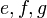$e,f,g$ for second fundamental form using this parametrization Fill this in later Fill this in later
principal curvatures using this parametrization eigenvalues of the shape operator Fill this in later
mean curvature arithmetic mean of the principal curvatures = half the trace of the shape operator Fill this in later
Gaussian curvature product of the principal curvatures = determinant of shape operator Fill this in later

## Verification of theorems

### Gauss-Bonnet theorem

Fill this in later